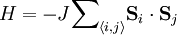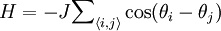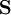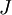# XY modelThis article is a 'stub' page, it has no, or next to no, content. It is here at the moment to help form part of the structure of SklogWiki. If you add sufficient material to this article then please remove the {{Stub-general}} template from this page.

The XY model, also known as the O(2) model because of its symmetry group, is a Heisenberg ferromagnetic with an easy-plane anisotropy. The Hamiltonian is given by$H = -J{\sum}_{\langle i,j\rangle}\mathbf{S}_i \cdot \mathbf{S}_{j}$

in other words$H=-J{\sum}_{\langle i,j\rangle}\cos(\theta_i-\theta_j)$

where the sum runs over all pairs of nearest neighbour spins,$\mathbf{S}$, and where$J$ is the coupling constant.

## XY universality class

(see: XY universality class)